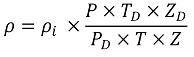# Stream Density Calculation (based on Observed Density)

### Stream Density Calculation (based on Observed Density)

#### This method is used to calculate the stream density from the observed density. For flow meters other than differential pressure flow meters the pressure and temperature at the flow meter is entered in the place of upstream pressure and temperature.

Upstream density is calculated as follows:Symbol Description Units
ρ Density at the upstream conditions kg/m3
ρi Observed density kg/m3
P Pressure at the upstream conditions bar a
PD Pressure at the flow meter bar a
T Temperature at the upstream conditions K
TD Temperature at the flow meter K
Z Compression factor at the upstream conditions -
ZD Compression factor corresponding with observed density -